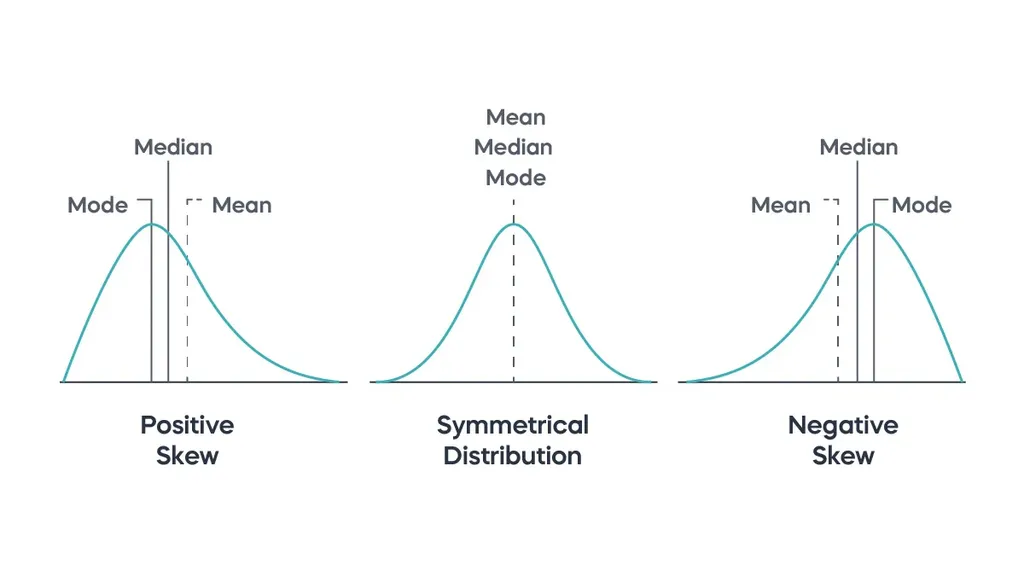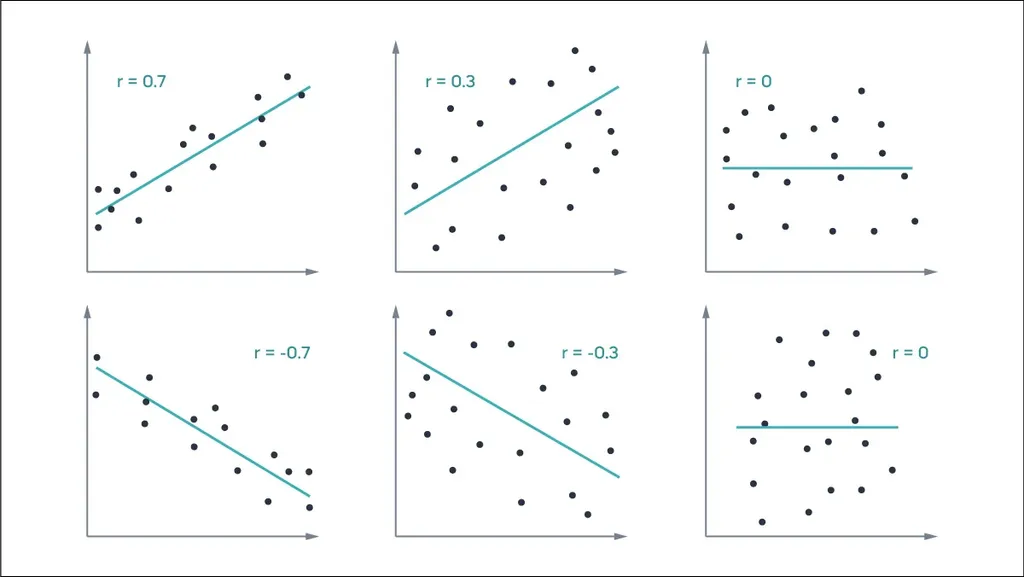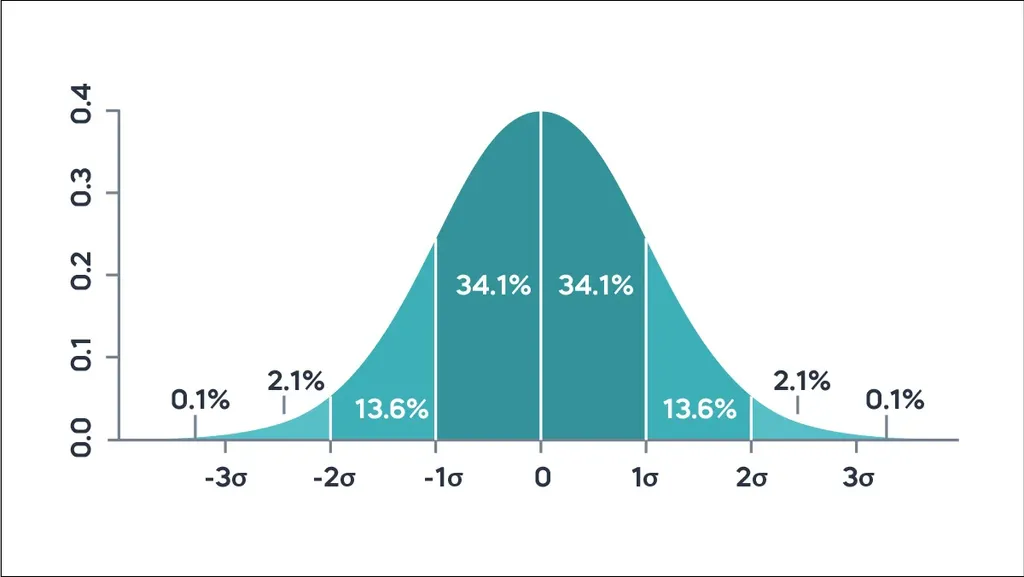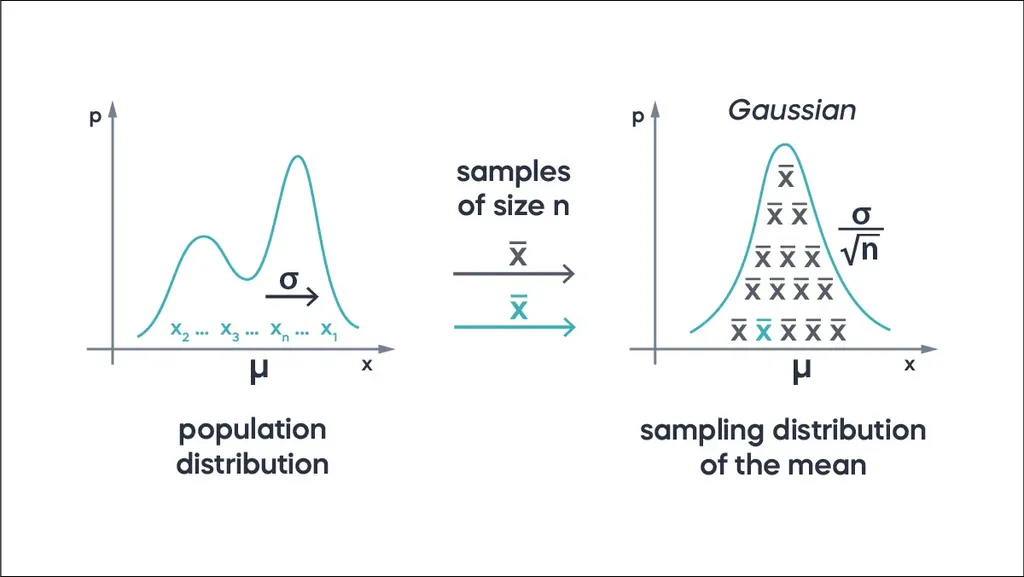# Top 15 Probability and Statistics Interview Questions for Data Scientists

Are you trying to prepare for a data science interview, but don’t you know where to start? It’s not just you; this task can be overwhelming. A data science interview may involve questions about anything from statistics and mathematics to deep learning and artificial intelligence.

So, it’s best to begin with the basics and gradually move on to more complex topics.

This article explains foundational concepts at the basis of machine learning and data analysis. More specifically, we present 15 common probability and statistics interview questions and answers to help you prepare for your data science or analytics job interview.

## 1. What Is the Difference Between Descriptive and Inferential Statistics?

Descriptive and inferential statistics are two different branches of the field. The former summarizes the characteristics and distribution of a dataset, such as mean, median, variance, etc. You can present those using tables and data visualization methods like box plots and histograms.

In contrast, inferential statistics allows you to formulate and test hypotheses for a sample and generalize the results to a broader population. Using confidence intervals, you can estimate the population parameters.

You must be able to explain the mechanisms behind these concepts because entry-level statistics questions for a data analyst interview often revolve around sampling, the generalizability of results, etc.

## 2. What Are the Main Measures Used to Describe the Central Tendency of Data?

Centrality measures are essential for exploratory data analysis. They indicate the center of the data distribution but yield different results. You must understand the difference between the main types to interpret and use them in analyses.

During a statistics job interview, you might need to explain the meaning of each measure of centrality, including mean, median, and mode:

• Mean (or average) is the sum of all observations divided by the total number of participants or cases (n).
• Median is the mid-point in a dataset ordered from the smallest to the largest when n is odd. With an even number of data points, it’s the average of the values in position n/2 and (n+1)/2—i.e., the two values in the middle.
• Mode is the most frequently appearing data point. It is a valuable measure when working with categorical variables.

## 3. What Are the Main Measures of Variability?

Variability measures are also crucial in describing data distribution. They show how spread-out data points are and how far away they are from the mean.

Some basic questions during a statistics interview might require you to explain the meaning and usage of variability measures. Here’s your cheat sheet:

• Variance measures the average squared distance of data points from the mean. A small variance corresponds to a narrow spread of the values, while a big variance implies that data points are far from the mean.
• Standard deviation is the square root of the variance. It shows the amount of variation of values in a dataset.
• Range is the difference between the maximum and minimum data value. It’s a good indicator of variability when there are no outliers in a dataset, but when there are, it can be misleading.
• Interquartile range (IQR) measures the spread of the middle part of a dataset. It’s essentially the difference between the third and the first quartile.

## 4. What Are Skewness and Kurtosis?

Next on our list of statistics questions for a data science interview are the measures of the shape of data distribution: skewness and kurtosis.

Skewness is an excellent way to measure the symmetry of distribution and the likelihood of a given value falling in the tails. With symmetrical distribution, the mean and median coincide. If the data distribution isn’t symmetrical, it’s skewed.

There are two types of skewness:

• Positive is when the right tail is longer. Most values are clustered around the left tail, and the median is smaller than the mean.
• Negative is when the left tail is longer. Most values are clustered around the right tail, and the median is greater than the mean.Kurtosis, on the other hand, reveals how heavy or light-tailed data is compared to the normal distribution. There are three types of kurtoses:

• Mesokurtic distributions approximate a normal distribution.
• Leptokurtic distributions have a pointy shape and heavy tails, indicating a high probability of extreme events occurring.
• Platykurtic distributions have a flat shape and light tails. They reveal a low probability of the occurrence of extreme events.

Knowing the meaning and calculations of these measures may be enough for an entry-level job. But statistics interview questions for advanced data science positions may revolve around using these concepts in practice.

If you wish to prepare for more advanced positions, try the 365 Data Scientist Career Track. It starts from the basics with statistics and probability, builds your knowledge with programming languages involved in machine learning and AI, such as SQL, and ends with portfolio, resume, and interview preparation lessons.

## 5. Describe the Difference Between Correlation and Autocorrelation

These two concepts tend to be confused, which makes it a good trick question for a statistics interview. To avoid surprises, we’ll explain the difference.

A correlation measures the linear relationship between two or more variables. It ranges between -1 and 1. It’s positive if the variables increase or decrease together. If it’s negative, one variable decreases while the other increases. When the value is 0, the variables aren’t related.

The following scatterplot illustrates the different types of correlation:In contrast, autocorrelation measures the linear relationship between two values of the same variable. Just like correlation, it can be positive or negative. Typically, we use it when we deal with a time series, i.e., different observations of the same construct.

## 6. Explain the Difference Between Probability Distribution and Sampling Distribution

As noted, you may be asked various statistics interview questions regarding sampling and the generalizability of results. The difference between probability and sampling distribution is just one example.

A probability distribution is a function used to calculate the probability of a random variable X taking different values. There are two main types depending on the variable: discrete and continuous. Examples of the former are the binomial and Poisson distributions, and of the latter: normal and uniform distributions.

A sampling distribution is the probability distribution of a statistic based on a range of random samples from a population. The definition sounds confusing, but it’s encountered often in practice.

For example, imagine you’re a clinical data analyst working on developing a new treatment for patients with Alzheimer’s. You’ll likely be working with samples from the entire population of individuals with the disease. So, you’ll use the sampling distribution during the data analysis.

## 7. What Is the Normal Distribution?

Normal distribution is a central concept in mathematics and data analysis. As such, it often appears in statistics interview questions.

The normal (or Gaussian) distribution is the most important probability distribution in statistics. It’s often called а “bell curve” because of its shape—tall in the middle, flat toward the ends.

A key characteristic of the normal distribution is that the mean and the median coincide. The mean is equal to 0, and the standard deviation is 1. With this information, we can calculate the following:

• 27% of the data falls within the +/-1 standard deviation of the mean.
• 45% of the data falls within +/-2 standard deviations of the mean.
• 7% of the data falls within +/-3 standard deviations of the mean.

This is known as the empirical rule.But what is so special about it?

It’s considered that naturally occurring phenomena have a normal distribution. As such, we often use it in data analysis to determine the probability of a data point being above or below a given value or for a sample mean being above or below the population mean.

## 8. What Are the Assumptions of Linear Regression?

Next, we move on from basic to intermediate probability and statistics interview questions. To further advance your knowledge on these topics, check out 365's Statistics course for data scientists.

But for now, let’s continue with linear regression, which is the basis of predictive analysis.

It investigates the relationship between one or more independent variables (predictors) and a dependent variable (outcome). More concretely, it examines the extent to which the independent variables are good predictors of the result.

The residual (or error term) equals the predictor variable minus the actual observed value. Linear regression models aim to find the "line of best fit” with minimal error.

The typical statistics interview questions for a data analyst job might involve the above definitions or the following four main assumptions that must be met to conduct linear regression analysis.

1. Linear relationship: A linear relationship exists between the predictors and the dependent variable.
2. Normality: The dependent variable has a normal distribution for any fixed value of the predictor.
3. Homoscedasticity: The variance of the error term is constant for every value of the independent variable.
4. Independence: All observations are independent—meaning there is no autocorrelation between the residuals.

## 9. What Is Hypothesis Testing?

We’ve already touched on this topic with some of the previous statistics and probability interview questions. But since it’s a fundamental part of data analysis, we wish to cover it in more detail.

Hypothesis testing allows us to evaluate a hypothesis about the population based on sample data. How do we conduct it?

First, we formulate a null hypothesis (or H0)—assuming no difference or relationship between the variables. For each null hypothesis, there’s an alternative one considering the opposite. If H0 is rejected, the alternative hypothesis is supported.

We need to choose an appropriate statistical test to determine whether the data supports a particular hypothesis. If the probability of the null hypothesis is below a predetermined significance level, we can reject it.

On that note, statistics questions for a data analyst interview may also regard different types of statistical tests. To help you prepare, we cover the basic ones.

## 10. What Are the Most Common Statistical Tests Used?

There are numerous statistical tests, each one serving a different purpose. Note the following common ones:

• The Shapiro-Wilk test is a statistical tool testing if a data distribution is normal.
• A t-test assesses whether the difference between two groups is statistically significant.
• Analysis of Variance (ANOVA) tests the statistical difference between more than two variables.

## 11. What Is the p-Value and How Do I Interpret It?

A p-value is the probability of obtaining given results if the null hypothesis is correct. To reject it, the p-value must be lower than a predetermined significance level α.

The most used significance level is 0.05. If the p-value is below 0.05, we can reject the null hypothesis and accept the alternative one.

In that case, the results are statistically significant.

This is a fundamental part of data analysis; therefore, a common statistics interview question.

## 12. What Is the Confidence Interval?

The confidence interval is the range within which we expect the results to lie if we repeat the experiment. It is the mean of the result plus and minus the predicted variation.

The standard error of the estimate determines the latter, while the interval's center coincides with the estimate's mean. The most common confidence interval is 95%.

## 13. What Are the Main Ideas of the Law of Large Numbers?

The Law of Large Numbers is a key theorem in probability and statistics with many practical applications in finance, business, etc. It states that if an experiment is repeated independently multiple times, the mean of all results will approximate the expected value.

A classic example is coin flipping. We know the probability (P) of getting tails is 50%. If the number of tails after 100 trials is X, then the expected value E(X) = n x P(X) = 100 x 0.5 = 50.

Let’s suppose we repeat the experiment multiple times.

The first time, we get X1= 65 tails; the second, X2 = 50 tails, and so on. Ultimately, we calculate the mean of all trials by adding the random variables (X1, X2, …, Xn) and dividing the sum by the number of experiments. Following the Law of Large Numbers, the mean of these results will approximate the expected value E(X) = 50.

This is a fundamental theorem in statistics with applications in machine learning; you can expect questions about it during a job interview.

## 14. What Is the Central Limit Theorem?

The Central Limit Theorem states that the distribution of sample means starts to resemble a normal distribution as the size of the sample increases. Interestingly, this happens even when the underlying population doesn’t have a Gaussian distribution.On the right, we see that—regardless of the population distribution—the sample means have a symmetrical bell shape distribution as the sample size increases. A sample size equal to or greater than 30 is typically considered large enough for the Central Limit Theorem to apply.

## 15. The Difference Between Probability and Likelihood

Lastly, we cover one of the fundamental principles of Bayesian statistics because data science interview questions may include that subject.

The difference between probability and likelihood is subtle but critical. Probability is the chance of a particular outcome to occur given the obtained values. When calculating it, we assume the parameters are trustworthy.

In contrast, likelihood aims to verify if the parameters in a model are trustworthy given the obtained results. In other words, we calculate the probability of a model being correct with the observed measurements.

## Probability and Statistics Interview Questions for Data Scientists: Next Steps

This concludes our list of probability and statistics interview questions and answers. We covered fundamental concepts to help you prepare for an interview and understand more complex data science and analytics topics.

If you desire to deepen your knowledge, try the 365 Data Science Program, which offers self-paced courses led by renowned industry experts. You'll learn from the basics to advanced specialization by executing many practical exercises and real-world business cases. If you wish to see how the training works, sign up below and access a selection of free lessons.

Learn data science with industry experts